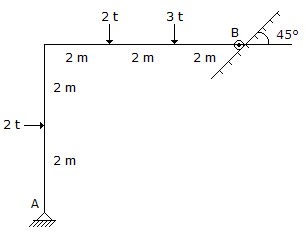# Civil Engineering - Applied Mechanics

### Exercise :: Applied Mechanics - Section 1

36.

The vertical reaction at the support A of the structure shown in below figure, isA. 1 t B. 2 t C. 3 t D. 2.5 t.

Explanation:

No answer description available for this question. Let us discuss.

37.

Pick up the correct statement from the following :

 A. Nature plays an important role in the launch of a satellite B. The earth's gravity reduces the speed of a satellite by 32 km per second C. The gravitational force relents as the satellite climbs higher D. The gravitational intensity declines with height E. All the above.

Explanation:

No answer description available for this question. Let us discuss.

38.

If the radius of the earth is 600 km the height of a mountain above sea level at the top of which a beat seconds pendulum at sea level, looses 27 seconds a day, is

 A. 500 metres B. 1000 metres C. 1500 metres D. 2000 metres E. 25000 metres.

Explanation:

No answer description available for this question. Let us discuss.

39.

The total time of collision and restitution of two bodies, is called

 A. time of collision B. period of collision C. period of impact D. all the above.

Explanation:

No answer description available for this question. Let us discuss.

40.

The length of a Second's pendulum, is

 A. 99.0 cm B. 99.4 cm C. 100 cm D. 101 cm E. 101.10 cm.